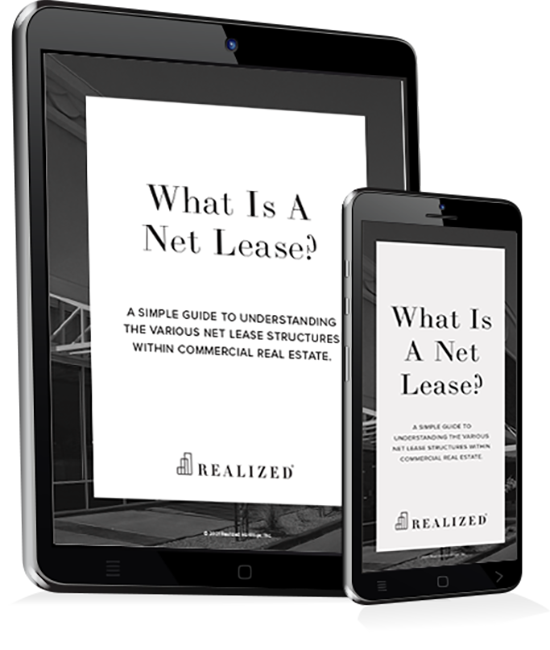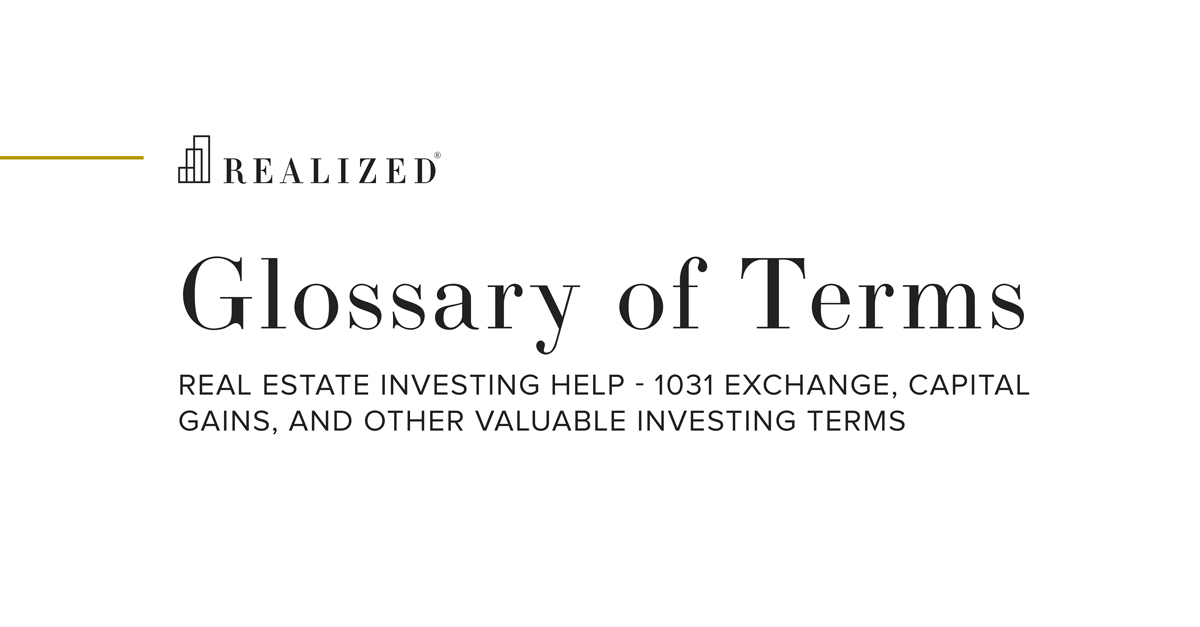Positive Leverage 2020-11-13 08:00:00

Positive leverage is when a business or individual borrows funds and then invests the funds at an interest rate higher than the rate at which they were borrowed.

The positive leverage calculation requires that you know the loan constant, which is the total annual loan payment (loan principal and interest) divided by the total loan. The calculation is:

Loan constant = [annual loan payment] / [total loan amount]

If the loan constant is greater than the cap rate, it is positive leverage. If it is lower than the cap rate, it is negative leverage.

As an example calculation, assume a property is acquired for \$1,000,000 and generates a net operating income (NOI) of \$60,000 resulting in a 6.0% unlevered cash-on-cash return prior to using any debt. In this case, the cap rate is also 6.0%.

If an investor is able to secure a 60% loan-to-value mortgage with an interest rate of 5.0% (interest-only), then total debt service payments would be \$30,000 (\$1,000,000 value times 60% LTV times 5.0%) and cash flow after debt service would be \$30,000 (\$60,000 NOI less \$30,000 debt service). Using debt, the investor would have contributed \$400,000 of equity (\$1,000,000 purchase price less \$600,000 mortgage) which results in increasing the cash-on-cash return to 10.0% (\$40,000 cash flow after debt service divided by \$400,000 equity). This 10.0% is higher than the 6.0% cap rate and results in positive leverage.

The levered scenario obviously has a better return than the unlevered scenario. But is there a point at which using leverage is no longer a viable option? Yes — a higher interest rate can create a negative leverage situation. As an example, an interest rate of 6.7% would create an annual debt payment of (\$600,0000 times 6.70%) \$40,200. \$60,000 NOI less \$40,200 debt service is \$19,800. Then \$19,800 divided by \$400,000 is 4.95%, which is less than the 6.0% cap rate, creating negative leverage.

### The Investor's Guidebook To Net Lease